Note: This is an archvied version of our old webpage. Some links might be broken. The current one can be found here.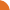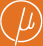Chair for Foundations of Software Reliability and Theoretical Computer Science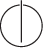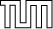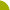Publications - Derivation Tree Analysis for Accelerated Fixed-Point ComputationReference:

Javier Esparza, Stefan Kiefer, and Michael Luttenberger. Derivation tree analysis for accelerated fixed-point computation. Theoretical Computer Science, 412(28):3226–3241, 2011.

Abstract:

We show that for several classes of idempotent semirings the least fixed-point of a polynomial system of equations X = f(X) is equal to the least fixed-point of a linear system obtained by ``linearizing'' the polynomials of f in a certain way. Our proofs rely on derivation tree analysis, a proof principle that combines methods from algebra, calculus, and formal language theory, and was first used in Esparza et al. (2007) to show that Newton's method over commutative and idempotent semirings converges in a linear number of steps. Our results lead to efficient generic algorithms for computing the least fixed-point. We use these algorithms to derive several consequences, including an O(N^3) algorithm for computing the throughput of a context-free grammar (obtained by speeding up the O(N^4) algorithm of Caucal et al. (2007), and a generalization of Courcelle's result stating that the downward-closed image of a context-free language is regular (Courcelle, 1992).

Suggested BibTeX entry:

@article{EKL11:TCS,
author = {Javier Esparza and Stefan Kiefer and Michael Luttenberger},
journal = {Theoretical Computer Science},
number = {28},
pages = {3226--3241},
title = {Derivation Tree Analysis for Accelerated Fixed-Point Computation},
volume = {412},
year = {2011}
}PDF (279 kB)Conference version